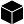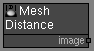# Mesh Distance node

Left Toolbar >Mesh Nodes >* Mesh Distance Node *

The Mesh Distance node renders the distance from the pixel in the mesh to a geometric entity in the 3D space. There are 4 geometric entities defined by points: Point, Line, Segment and Plane. The Mesh Distance node generates a VALUE image with the distance of each pixel point to the 3D entity.

modelsModel

outputimage## Procedure

### To create a Mesh Distance image:

1. Load one of more model in the Scene Window.

2. On the left toolbar choose Mesh Nodes > Mesh Distance.>3. Drag the Mesh Distance icon into the Node Graph.4. Connect the output socket into the image socket in the Mesh Distance node.

5. Adjust the attributes of the Mesh Distance node to modify it.

## Outputs

image
A monochormatic image with the distance from the pixel to the Mesh entity.

## Attributes Panel*Position * Position X and Y of the output image.

Size Size X and Y of the output image.

Method Defines the geometric entity.
to point a: Distance from the pixel in the mesh to the Point A.
to point b: Distance from the pixel in the mesh to the Point B.
to line: Distance from the pixel in the mesh to the line defined by points A and B.
to segment: Distance from the pixel in the mesh to the line segment defined by points A and B.
to plane: Distance from the pixel in the mesh to the plane defined by point A and the normal B - A.

Point A X The x coordinate of Point A.

Point A Y The y coordinate of Point A.

Point A Z The z coordinate of Point A.

Point B X The x coordinate of Point B.

Point B Y The y coordinate of Point B.

Point B Z The y coordinate of Point B.

Padding The size of the region of pixels around the rendered components.

### Deprecated Attributes

The Radius and Falloff attributes have been deprecated. The Mesh Distance node now returns the absolute distance of each pixel surface point to the 3D entity. To achieve a similar effect use a Value Range node.

## Samples

The following examples were rendered using a 200 x 200 Plane model, and two points at A (-50,-50,0) and B (50, 50, 0).

to point a:to point b:to line:to segment:to plane: